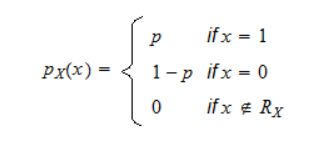Show Question
Bernoulli Distribution

The bernoulli distribution is a distribution over a single binary random variable.

Suppose you perform an experiment with two possible outcomes: either success or failure. Success happens with probability p, while failure happens with probability 1-p. A random variable that takes value 1 in case of success and 0 in case of failure is called a Bernoulli random variable (alternatively, it is said to have a Bernoulli distribution).

Let$p \varepsilon (0, 1)$. We say that X has a Bernoulli distribution with parameter p if its probability mass function is:A random variable having a Bernoulli distribution is also called a Bernoulli random variable.

Expected value and variance:
E[X] = p
Var[X] = p(1-p)

A sum of independent Bernoulli random variables is a binomial random variable.